Question

# - You may assume that the per capita consumption of bottled water is approx. normally distributed...

- You may assume that the per capita consumption of bottled water is approx. normally distributed with a mean of 32.1 and a standard deviation of 11 gallons. Answer the following:

a. What is the probability that someone consumes exactly 15 gallons of water?

b. What is the probability that someone consumed between 30 and 40 gallons of water?

c. 99.5% of people consumed less than how many gallons of water?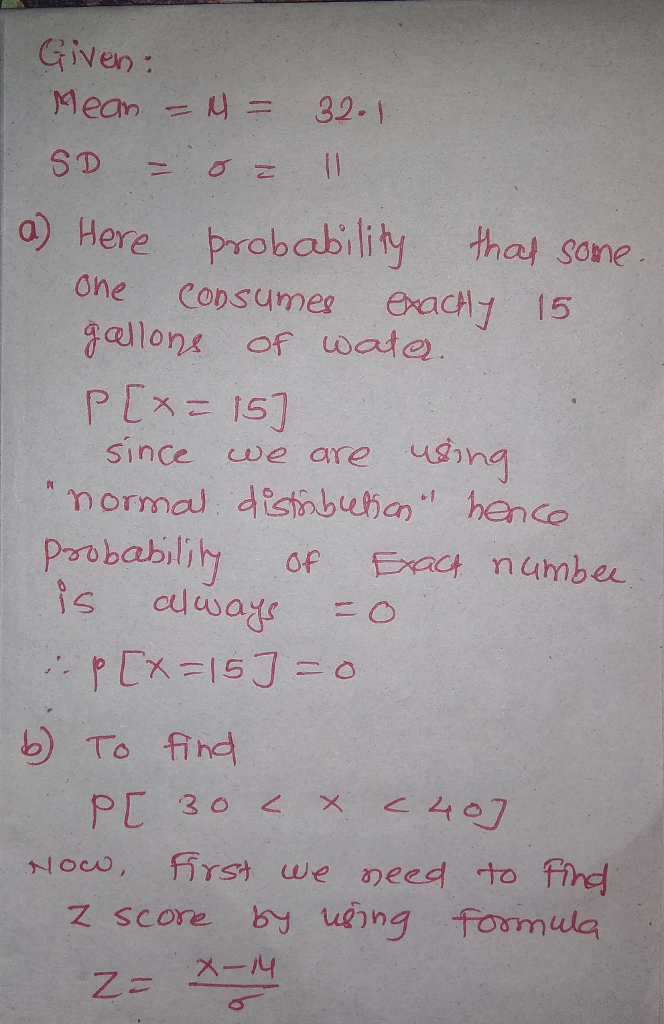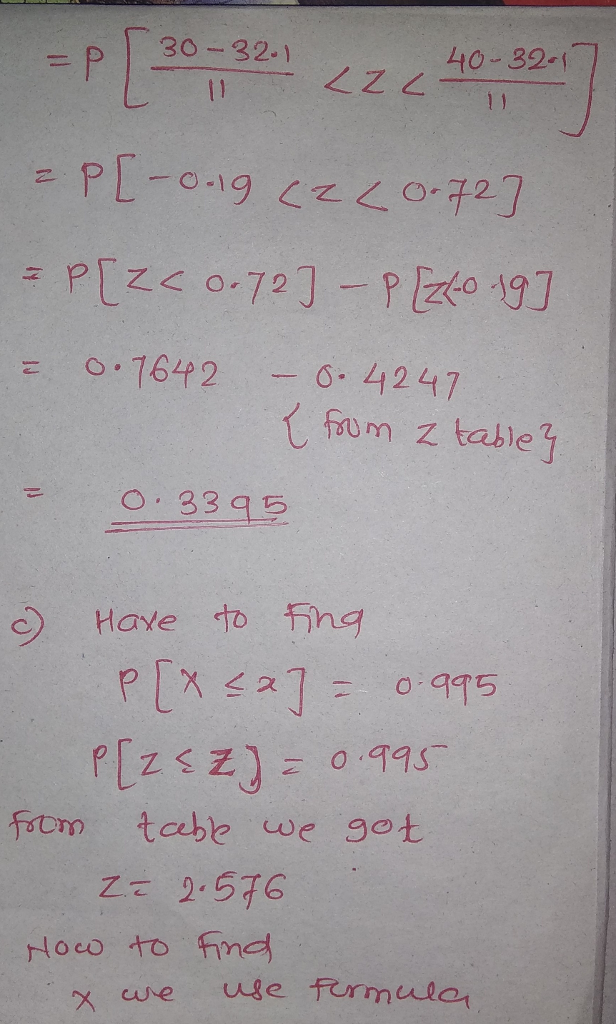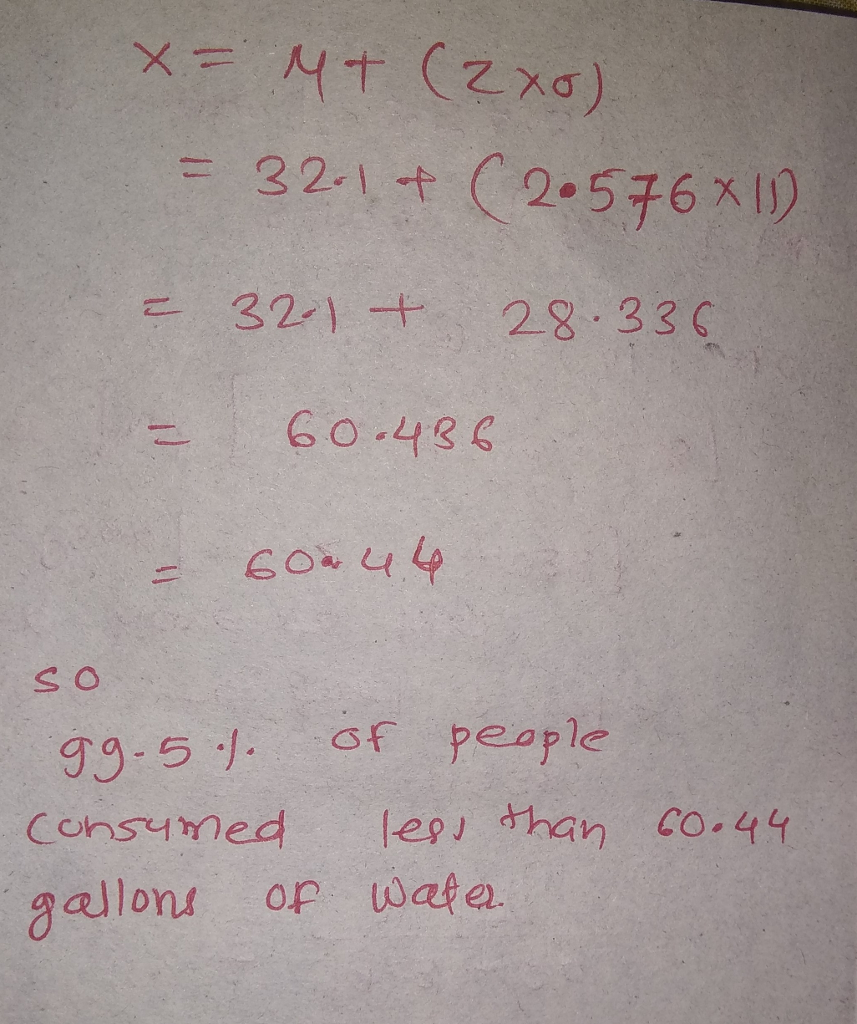#### Earn Coins

Coins can be redeemed for fabulous gifts.

Similar Homework Help Questions
• ### The annual per capita consumption of bottled water was 30.3 gallons. Assume that the per capita...

The annual per capita consumption of bottled water was 30.3 gallons. Assume that the per capita consumption of bottled water is approximately normally distributed with a mean of 30.3 and a standard deviation of 10 gallons. a. What is the probability that someone consumed more than 40 gallons of bottled​ water? b. What is the probability that someone consumed between 20 and 30 gallons of bottled​ water? c. What is the probability that someone consumed less than 20 gallons of...

• ### In​ 2008, the per capita consumption of soft drinks in Country A was reported to be...

In​ 2008, the per capita consumption of soft drinks in Country A was reported to be 19.04 gallons. Assume that the per capita consumption of soft drinks in Country A is approximately normally​ distributed, with a mean of 19.04 gallons and a standard deviation of 5 gallons. Complete parts​ (a) through​ (d) below. a. What is the probability that someone in Country A consumed more than 11 gallons of soft drinks in​ 2008? ​(Round to four decimal places as​ needed.)...

• ### In 2008, the per capita consumption of soft drinks in Country A was reported to be...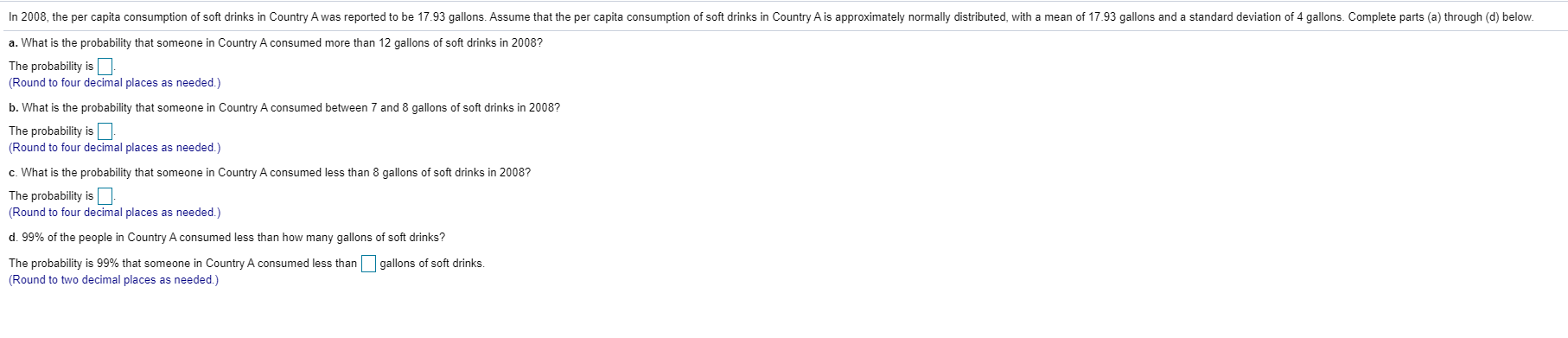In 2008, the per capita consumption of soft drinks in Country A was reported to be 17.93 gallons. Assume that the per capita consumption of soft drinks in Country A is approximately normally distributed, with a mean of 17.93 gallons and a standard deviation of 4 gallons. Complete parts (a) through (d) below. a. What is the probability that someone in Country A consumed more than 12 gallons of soft drinks in 2008? The probability is (Round to four decimal...

• ### In​ 2008, the per capita consumption of soft drinks in Country A was reported to be...

In​ 2008, the per capita consumption of soft drinks in Country A was reported to be 18.51 gallons. Assume that the per capita consumption of soft drinks in Country A is approximately normally​ distributed, with a mean of 18.51 gallons and a standard deviation of 5 gallons. Complete parts​ (a) through​ (d) below. a. What is the probability that someone in Country A consumed more than 13 gallons of soft drinks in​ 2008? ​(Round to four decimal places as​ needed.)...

• ### In 2008, the per capita consumption of soft drinks in Country Awas reported to be 18.95...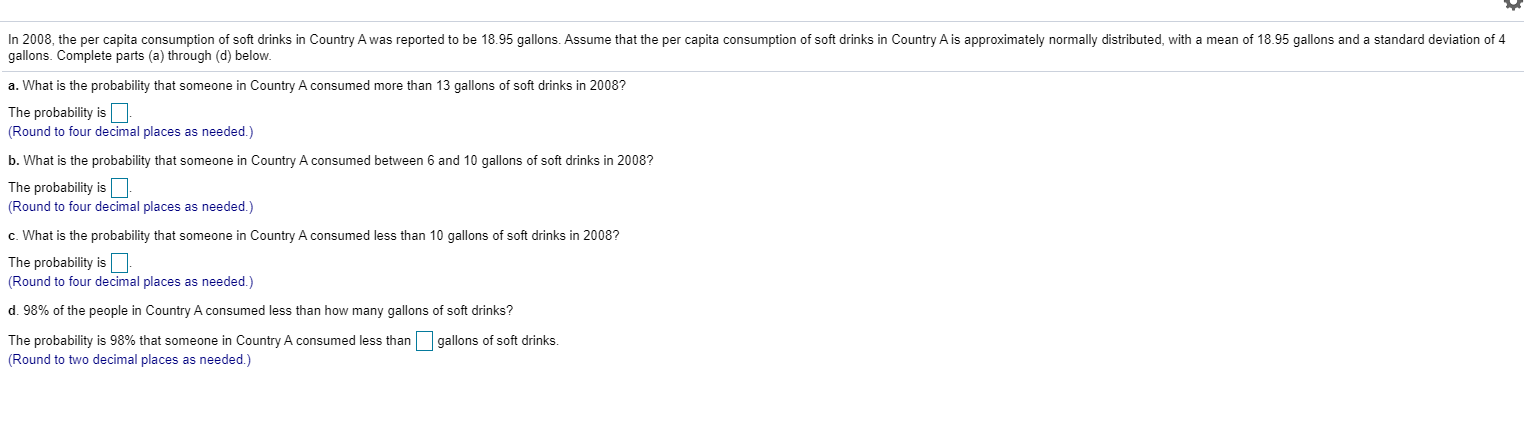In 2008, the per capita consumption of soft drinks in Country Awas reported to be 18.95 gallons. Assume that the per capita consumption of soft drinks in Country A is approximately normally distributed, with a mean of 18.95 gallons and a standard deviation of 4 gallons. Complete parts (a) through (d) below. a. What is the probability that someone in Country A consumed more than 13 gallons of soft drinks in 2008? The probability is (Round to four decimal places...

• ### In 2008, the per capita consumption of soft drinks in Country A was reported to be...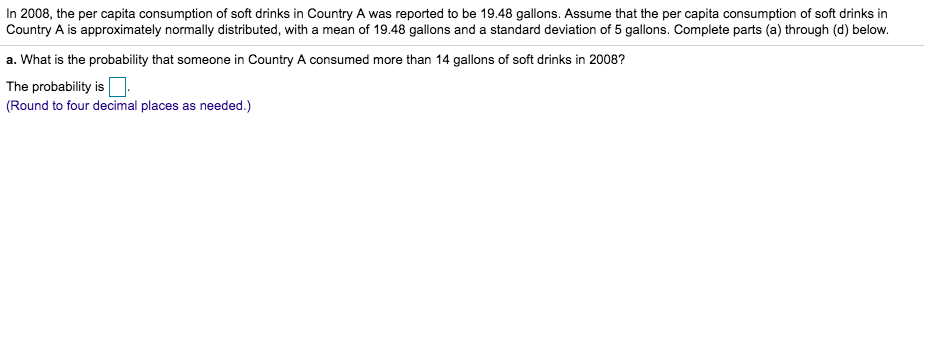In 2008, the per capita consumption of soft drinks in Country A was reported to be 19.48 gallons. Assume that the per capita consumption of soft drinks in Country A is approximately normally distributed, with a mean of 19.48 gallons and a standard deviation of 5 gallons. Complete parts (a) through (d) belovw a. What is the probability that someone in Country A consumed more than 14 gallons of soft drinks in 2008? The probability is (Round to four decimal...

• ### Please Type your answer: In 2018, the average water consumption per capita in Inland X for the year was 93.5 gallons. Assume that the water consumption per capita in Inland X follows a normal and symmetrical distribution with a mean of 93.5 gallons and a

1.     In 2018, the average water consumption per capita in Inland X for the year was 93.5 gallons. Assume that the water consumption per capita in Inland X follows a normal and symmetrical distribution with a mean of 93.5 gallons and a standard deviation of 12 gallons. Find the following:a.     90% of the people in Inland X consumed more than how many gallons of water?

• ### The per capita consumption of bottled water was 7 gallons in 1990 and has been increasing...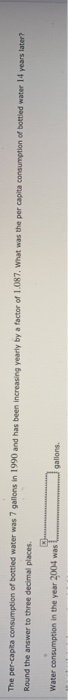The per capita consumption of bottled water was 7 gallons in 1990 and has been increasing yearly by a factor of 1.087. What was the per capita consumption of bottled water 14 years later? Round the answer to three decimal places. Water consumption in the year 2004 was gallons

• ### 1. The amount of soda in a 12 ounce bottle is supposed to be 12 ounces,...

1. The amount of soda in a 12 ounce bottle is supposed to be 12 ounces, right? There is some variability in the amount that the machines dispense into the bottles. Let the real amount of soda in each bottle follow a Normal distribution with mean 12.2 and standard deviation 0.3. a. If the bottle can only hold 13 ounces, what is the probability the bottle will overflow? In other words, what is the probability that the machine dispenses more...

• ### suppose the lengths of the pregnancies' of a certain animal are approx. normally distributed with a...

suppose the lengths of the pregnancies' of a certain animal are approx. normally distributed with a mean = 255 days and standard deviation of 14 days what is the probability that a random sample of 48 pregnancies has a mean gestation of 250 days or less? (d) the probability that the mean of a random sample of 48 pregnancies is less than 250 days is approx. ___?